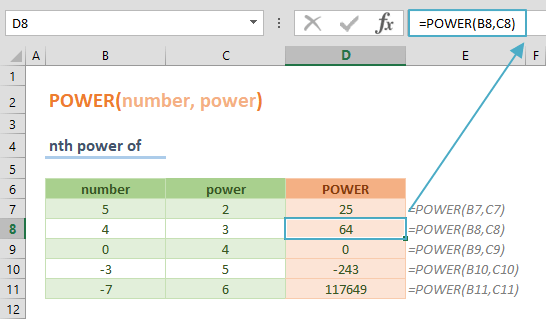POWER is a Math and Trigonometry function that can calculate and returns the result of a number raised to a specified power. For other methods and finding different exponents, please see: How to find square root in Excel. In this guide, we’re going to show you how to use the Excel POWER function and also go over some tips and error handling methods.

## Supported versions

• All Excel versions

## POWER Function Syntax

POWER(number, power)

## Arguments

 number The numeric value which you want to raise to a power. power The numeric exponent value to raise power to.

## Examples

### Calculating the nth power of a number

The function requires the number and the power arguments as input values. The number argument represents the base of the exponent operation. Thus, the POWER executes the xy (x to the power of y) operation. For example, to calculate 4, raised to the power of 3, use the following function:

=POWER(4,3)### Calculating the nth root of a number

You can use the POWER function to calculate the nth root of a number. You can get the root of a number if you raise the number with a rational exponent.As a result, using a rational exponent for the power argument gives the root of the specified number. For example, to calculate the cube root of 16, you can use a formula like below.=POWER(16,1/3)

## Tips

• Use rational exponents (1/y) for the power argument to calculate nth root of a number using the POWER function.
• Alternatively, you can use caret symbol (^) to find the result when a number is raised to the power. If the base is x and the power is y, the formula would be

=x^y